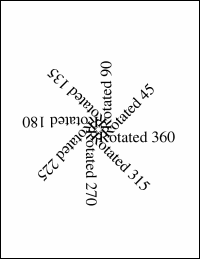Rotate MethodRotates about a locked anchor point (angle in degrees).Syntax

 `XTransform.Rotate Angle, AnchorX, AnchorY`Params

 Name Type Description Angle Double The angle to rotate in degrees. AnchorX Double The horizontal coordinate about which the rotation should be applied. AnchorY Double The vertical coordinate about which the rotation should be applied.Notes

 This method rotates the world space about a locked anchor point. The angle is specified in degrees anti-clockwise.Example

 Here, we add a number of chunks of text rotated at different angles about the middle of the document. ```Set theDoc = Server.CreateObject("ABCpdf12.Doc") theDoc.FontSize = 48 theDoc.TextStyle.Indent = 48 For i = 1 To 8   theAngle = i * 45   theDoc.Pos = "302 396"   theDoc.Transform.Reset   theDoc.Transform.Rotate theAngle, 302, 396   theDoc.AddText "Rotated " & theAngle Next theDoc.Save "c:\mypdfs\transformrotate.pdf"```transformrotate.pdf